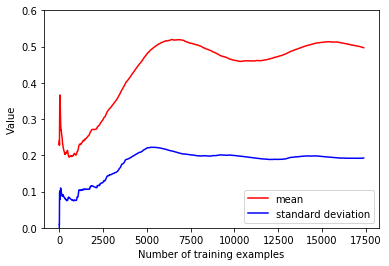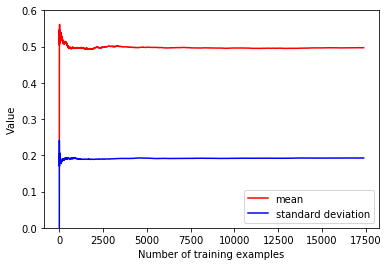sklearn 是 python 中一个非常著名的机器学习库，但是一般都是在单机上使用而不支持分布式计算，因此往往跟大规模的机器学习扯不上关系。这里通过 sklearn 进行的大规模机器学习指的也不是分布式机器学习，而是指当数据量比内存要大时怎么通过 sklearn 进行机器学习，更准确来说是 out-of-core learning， 这里涉及到的一个核心思想是将数据转化为流式输入，然后通过 SGD 更新模型的参数，当然其中还涉及到一些其他的细节和trick，下面会详细描述。

## sklearn 中的 SGD

• loss : 表示具体的分类器，可选的值为 hinge、log、modified_huber、squared_hinge、perceptron；如 hinge 表示 SVM 分类器，log 表示logistics regression等
• penalty：正则项，用于防止过拟合(默认为 L2 正则项)
• learning_rate: 表示选择哪种学习速率方案，共有三种：constant、optimal、invscaling，各种详细含义可参考官方文档

## 流式数据中的特征工程

### feature scaling

$$\sigma^2 = \frac{1}{n} \sum_x(x-\mu)^2$$

$$\sigma’^2 = \frac{1}{n} \sum_x(x^2 - 2x\mu’ + \mu’^2) = \frac{1}{n} \sum_x(x^2) - \frac{1}{n}(2n\mu’^2 - n\mu’^2) = \frac{1}{n} \sum_x(x^2) - \mu’^2$$shuffle 后的文件### hasing trick

hahsing trick 利用了 hash 函数，通过hash后取模，将样本的值映射到预先定义好的固定长度的槽列中的某个槽中，这种方法需要对 categorical feature 的所有可能取值有大概的估计，而且可能会出现冲突的情况，但是如果对categorical feature 的所有可能取值有较准确的估计时，冲突的概率会比较低。下面是利用 sklearn 中的 HashingVectorizer 进行这种编码的一个例子